Test: Propositional & First Order Logic- 1

# Test: Propositional & First Order Logic- 1 - Computer Science Engineering (CSE)

Test Description

## 20 Questions MCQ Test GATE Computer Science Engineering(CSE) 2023 Mock Test Series - Test: Propositional & First Order Logic- 1

Test: Propositional & First Order Logic- 1 for Computer Science Engineering (CSE) 2023 is part of GATE Computer Science Engineering(CSE) 2023 Mock Test Series preparation. The Test: Propositional & First Order Logic- 1 questions and answers have been prepared according to the Computer Science Engineering (CSE) exam syllabus.The Test: Propositional & First Order Logic- 1 MCQs are made for Computer Science Engineering (CSE) 2023 Exam. Find important definitions, questions, notes, meanings, examples, exercises, MCQs and online tests for Test: Propositional & First Order Logic- 1 below.
Solutions of Test: Propositional & First Order Logic- 1 questions in English are available as part of our GATE Computer Science Engineering(CSE) 2023 Mock Test Series for Computer Science Engineering (CSE) & Test: Propositional & First Order Logic- 1 solutions in Hindi for GATE Computer Science Engineering(CSE) 2023 Mock Test Series course. Download more important topics, notes, lectures and mock test series for Computer Science Engineering (CSE) Exam by signing up for free. Attempt Test: Propositional & First Order Logic- 1 | 20 questions in 60 minutes | Mock test for Computer Science Engineering (CSE) preparation | Free important questions MCQ to study GATE Computer Science Engineering(CSE) 2023 Mock Test Series for Computer Science Engineering (CSE) Exam | Download free PDF with solutions
 1 Crore+ students have signed up on EduRev. Have you?
Test: Propositional & First Order Logic- 1 - Question 1

### What is the logical translation of the following statement? "None of my friends are perfect."

Detailed Solution for Test: Propositional & First Order Logic- 1 - Question 1

F(x) ==> x is my friend
P(x) ==> x is perfect

A. There exist some friends which are not perfect

B. There are some people who are not my friend and are perfect

C. There exist some people who are not my friend and are not perfect.

D. There doesn't exist any person who is my friend and perfect

Test: Propositional & First Order Logic- 1 - Question 2

### Let # be a binary operator defined as X # Y = X′ + Y′ where X and Y are Boolean variables. Consider the following two statements. S1: (P # Q) # R = P # (Q # R) S2: Q # R = R # Q Which of the following is/are true for the Boolean variables P, Q and R?

Detailed Solution for Test: Propositional & First Order Logic- 1 - Question 2

S2 is true, as X' + Y' = Y' + X'

S1 is false.
Let P = 1, Q = 1, R = 0, we get different results
(P # Q) # R = (P' + Q')' + R' = (0 + 0)' + 1 = 1 + 1 = 1
P # (Q # R) = P' + (Q' + R')' = 0 + (0 + 1)' = 0 + 0 = 0

Test: Propositional & First Order Logic- 1 - Question 3

### What is the correct translation of the following statement into mathematical logic? “Some real numbers are rational”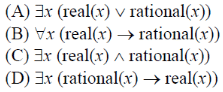Test: Propositional & First Order Logic- 1 - Question 4

Which one of the following options is CORRECT given three positive integers x, y and z, and a predicate?

P(x) = ¬(x=1)∧∀y(∃z(x=y*z)⇒(y=x)∨(y=1))

Detailed Solution for Test: Propositional & First Order Logic- 1 - Question 4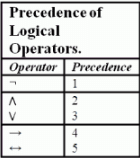So the predicate is evaluated asP(x) = (¬(x=1))∧(∀y(∃z(x=y*z)⇒((y=x)∨(y=1))) P(x) being true means x ≠ 1 and For all y if there exists a z such that x = y*z then y must be x (i.e. z=1) or y must be 1 (i.e. z=x) It means that x have only two factors first is 1 and second is x itself. This predicate defines the prime number.

Test: Propositional & First Order Logic- 1 - Question 5

Suppose the predicate F(x, y, t) is used to represent the statement that person x can fool person y at time t. which one of the statements below expresses best the meaning of the formula ∀x∃y∃t(¬F(x, y, t))?

Detailed Solution for Test: Propositional & First Order Logic- 1 - Question 5

∀ x ∃ y ∃ t(¬ F(x,y,t)) => ∀ x ¬(∀ y ∀ t F(x,y,t))

Test: Propositional & First Order Logic- 1 - Question 6

Which one of the following is the most appropriate logical formula to represent the statement? "Gold and silver ornaments are precious". The following notations are used: G(x): x is a gold ornament S(x): x is a silver ornament P(x): x is precious

Detailed Solution for Test: Propositional & First Order Logic- 1 - Question 6

=> This statement can be expressed as => For all X, x can be either gold or silver then the ornament X is precious => For all X, (G(X) v S(x)) => P(X).

Test: Propositional & First Order Logic- 1 - Question 7

In propositional logic P ↔ Q is equivalent to (Where ~ denotes NOT):

Detailed Solution for Test: Propositional & First Order Logic- 1 - Question 7

P ↔ Q = (P → Q) ∧ (Q → P)
P ↔ Q = (~P ∨ Q) ∧ (~Q v P)
So, option (B) is correct.

Test: Propositional & First Order Logic- 1 - Question 8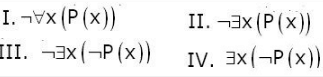Q. Which of the above two are equivalent?

Detailed Solution for Test: Propositional & First Order Logic- 1 - Question 8

According to negation property of universal qualifier and existential quantifier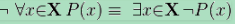Test: Propositional & First Order Logic- 1 - Question 9

Let fsa and pda be two predicates such that fsa(x) means x is a finite state automaton, and pda(y) means that y is a pushdown automaton. Let equivalent be another predicate such that equivalent (a, b) means a and b are equivalent. Which of the following first order logic statements represents the following: Each finite state automaton has an equivalent pushdown automaton.

Detailed Solution for Test: Propositional & First Order Logic- 1 - Question 9

Considering each option :
(A) If everything is a FSA, then there exists an equivalent PDA for everything.
(B) It is not the case that for all y if there exist a FSA then it has an equivalent PDA.
(C) Everything is a FSA and has an equivalent PDA.
(D) Everything is a PDA and has exist an equivalent FSA.
Thus, option (A) is correct.

Please comment below if you find anything wrong in the above post.

Test: Propositional & First Order Logic- 1 - Question 10

P and Q are two propositions. Which of the following logical expressions are equivalent?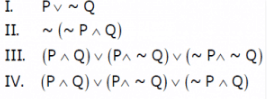Detailed Solution for Test: Propositional & First Order Logic- 1 - Question 10

I and II are same by Demorgan's law The IIIrd can be simplified to I.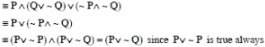Test: Propositional & First Order Logic- 1 - Question 11

Let Graph(x) be a predicate which denotes that x is a graph. Let Connected(x) be a predicate which denotes that x is connected. Which of the following first order logic sentences DOES NOT represent the statement: “Not every graph is connected”?

Detailed Solution for Test: Propositional & First Order Logic- 1 - Question 11

Option A and option C are same G(x)-->C(x) can also be written as ~G(x) or C(x), and they are the correct representation. Option C says :There exists a graph and graph is not connected ,which is equivalent to given sentence. Option D: Every x which is graph is not connected. Option D is correct choice

Test: Propositional & First Order Logic- 1 - Question 12

Which of the following is TRUE about formulae in Conjunctive Normal Form?

Detailed Solution for Test: Propositional & First Order Logic- 1 - Question 12

We can easily prove that for any formula, there is a truth assignment for which at least half the clauses evaluate to true . Proof : Consider an arbitrary truth assignment. For each of its clause ‘j’ , introduce a random variable. Xj = 1 if clause ‘j’ is satisfied Xj = 0 otherwise Then, X = summation of (j * Xj) is the number of satisfied clauses. Given any clause ’c’ , it is unsatisfied only if all of its ‘k’ constituent literals evaluates to false as they are joined by OR operator. Now, because each literal within a clause has a 1/2 chance of evaluating to true independently of any of the truth value of any of the other literals, the probability that they are all false is (1 / 2)k . Thus, the probability that ‘c’ is satisfied = 1 − (1 / 2)k So, E(Xj) = 1 * (1 / 2)k = (1 / 2)k Therefore, E(Xj) >= 1/2 Summation on both sides to get E(X). Therefore, we have E(X) = summation of (j * Xj) >= m/2 where ‘m’ is the number of clauses. E(X) represents expected number of satisfied clauses. Thus, there must exist an assignment that satisfies at least half of the clauses. Please comment below if you find anything wrong in the above post.

Test: Propositional & First Order Logic- 1 - Question 13

The following propositional statement is (P → (Q v R)) → ((P ^ Q) → R)

Detailed Solution for Test: Propositional & First Order Logic- 1 - Question 13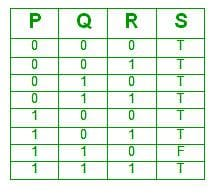A formula is satisfiable if there is atleast one assignment for which it is true. Clearly this formula is satisfiable as there are 7 assignments for which it is true.
A formula is valid if it is true for all assignments, which is not the case here.
Thus, option (B) is correct.

Test: Propositional & First Order Logic- 1 - Question 14

Which one of the following Boolean expressions is NOT a tautology?

Detailed Solution for Test: Propositional & First Order Logic- 1 - Question 14

(A) a -> b means if ‘a’ is true then ‘b’ is also true. Now, ‘b’ is true. Therefore, ‘c’ is also true. => Using transitivity rule , a -> c
(B) a <-> c is true if both ‘a’ and ‘c’ have same values. Let ‘a’, ‘b’ and c’ be false. Expression 'a and b' is false and expression 'not b' is true. RHS of the given equation should be true. But it evaluates to be false. Therefore, contradiction is there.
Option (B) is not a tautology.
(C) Let ‘a’ and ‘c’ be false. ’b’ be true a and b and c is false c or a is false
(D) Let ‘b’ be true. b -> a means if ‘b’ is true then ‘a’ is also true. Therefore, ‘a’ and ‘b’ both evaluates to be true.

Thus, option (B) is correct.

Please comment below if you find anything wrong in the above post.

Test: Propositional & First Order Logic- 1 - Question 15

The CORRECT formula for the sentence, “not all rainy days are cold” is

Detailed Solution for Test: Propositional & First Order Logic- 1 - Question 15

(A) Note that (p ∧ ~q) ≡ ~(p -> q). So it means rainy day to cold implication is false for all days. Which means non-rainy days are cold. (B) For all days, if day is not rainy, then it is cold [Non-Rainy days are cold] (C) There exist some days for which not rainy implies cold. [Some non-rainy days are cold] (D) Note that (p ∧ ~q) ≡ ~(p -> q). So it means rainy day to cold implication is false for some days. Which means not all rainy days are cold.

Test: Propositional & First Order Logic- 1 - Question 16

Which one of the first order predicate calculus statements given below correctly express the following English statement?

Tigers and lions attack if they are hungry or threatened.

Detailed Solution for Test: Propositional & First Order Logic- 1 - Question 16

The statement "Tigers and lions attack if they are hungry or threatened" means that if an animal is either tiger or lion, then if it is hungry or threatened, it will attack. So option (D) is correct. Don't get confused by "and" between tigers and lions in the statement. This "and" doesn't mean that we will write "tiger(x) ∧ lion(x) ", because that would have meant that an animal is both tiger and lion, which is not what we want.

Test: Propositional & First Order Logic- 1 - Question 17

Consider the following propositional statements:
P1 : ((A ∧ B) → C)) ≡ ((A → C) ∧ (B → C))
P2 : ((A ∨ B) → C)) ≡ ((A → C) ∨ (B → C))
which one of the following is true?

Detailed Solution for Test: Propositional & First Order Logic- 1 - Question 17

The easiest way to solve this question by creating truth tables for the expressions given. Note that P1 will be a tautology if truth table for left expression is exactly same as truth table for right expression. Same holds for P2 also.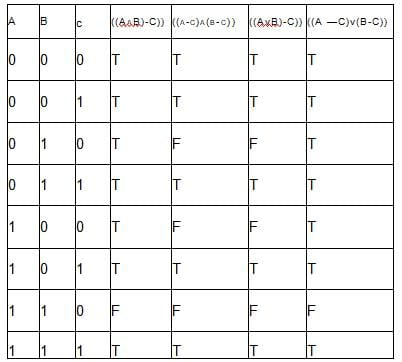So as we see from table, none of the P1 or P2 are tautologies, so option (D) is correct.

Test: Propositional & First Order Logic- 1 - Question 18

A logical binary relation □ ,is defined as follows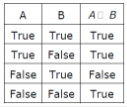Q. Let ~ be the unary negation (NOT) operator, with higher precedence than □. Which one of the following is equivalent to A∧B ?

Detailed Solution for Test: Propositional & First Order Logic- 1 - Question 18

In A∧B, we have 3 entries as False, and one as True. In table, it is opposite case, so we have to negate A □ B, moreover, we want True only when both A and B are true, so in 3rd entry (which becomes true after negation), we want both true, so we have to negate A also. So A ∧ B ≡ ~(~A □ B), so option (D) is correct.

Test: Propositional & First Order Logic- 1 - Question 19

Let P, Q and R be three atomic prepositional assertions. Let X denote (P v Q) → R and Y denote (P → R) v (Q → R). Which one of the following is a tautology?

Detailed Solution for Test: Propositional & First Order Logic- 1 - Question 19

X = (P ⋁ Q) → R
= ~(P ⋁ Q) ⋁ R

= (~P ⋀ ~Q) ⋁ R

= (~P ⋁ R) ⋀ (~Q ⋁ R)

= (P → R) ⋀ (Q → R)

X → Y is true as (A ⋀ B) → (A ⋁ B) is always TRUE but reverse implication is not always true.

Test: Propositional & First Order Logic- 1 - Question 20

What is the first order predicate calculus statement equivalent to the following?

Every teacher is liked by some student

## GATE Computer Science Engineering(CSE) 2023 Mock Test Series

151 docs|215 tests
Information about Test: Propositional & First Order Logic- 1 Page
In this test you can find the Exam questions for Test: Propositional & First Order Logic- 1 solved & explained in the simplest way possible. Besides giving Questions and answers for Test: Propositional & First Order Logic- 1, EduRev gives you an ample number of Online tests for practice

## GATE Computer Science Engineering(CSE) 2023 Mock Test Series

151 docs|215 tests(Scan QR code)